Next: Constraint Propagation Up: Programming with a Constraint Previous: Primitive Constraints

## CLP(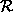)

The constraint logic programming scheme, written CLP(X), is a generic extension of logic programming to compute over any given constraint store. Logic programming over a constraint store has all the advantages of traditional logic programming, plus many further advantages for high-level modelling and efficient evaluation. If the constraint store holds primitive constraints from the class X, logic programming over this constraint store is termed CLP(X). In this section we shall use a particular class of primitive constraints, linear equations and inequations, termed, to illustrate the scheme. We shall use an example from

(Colmerauer, 90) to illustrate how it works.

Given the definition of a meal as consisting of an appetiser, a main meal and a dessert, and given a database of foods and their calorific values, we wish to construct light meals i.e. meals whose total calorific value does not exceed 10.

Aprogram for solving this problem is shown in figure 3.2.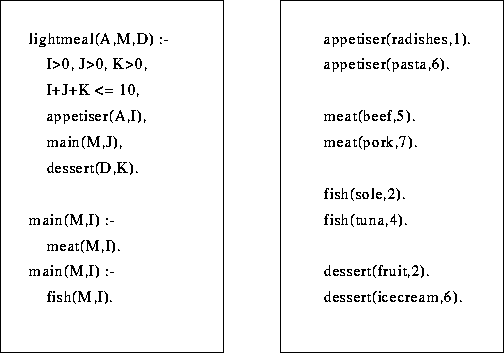Figure 1: The Lightmeal Program inAprogram is syntactically a collection of clauses which are either rules or facts. Rules are as in logic programming, with the addition that they can include constraints, such as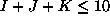, in their bodies.

The intermediate results of the execution of this program will be descibed as computation states. Each such state comprises two components, the constraint store, and the remaining goals to be solved. We shall represent such a state as `Store @ Goals`.programs are executed by reducing the goals using the program clauses. Consider the query `lightmeal(X,Y,Z).` which asks for any way of putting together a light meal. The initial state has an empty constraint store and one goal: ` @ lightmeal(X,Y,Z)`.

This goal is reduced using the clause whose head matches the goal. The goal is then replaced by the body of the clause, adding any constraints to the constraint store:

```X=A,Y=M,Z=D, I+J+K =< 10, I>=0, J>=0, K>=0 @
appetiser(A,I), main(M,J), dessert(D,K)```

The execution continues, choosing a matching clause for each goal and using it to reduce the goal. Variables which neither appear in the original goal, nor any of the currently remaining goals are projected out, as described above. A successful derivation is a sequence of such steps that reduces all the goals without ever meeting an inconsistency on adding constraints to the store. An example is:

```X=radishes, Y=M, Z=D, 1+J+K=<10, J>=0, K>=0 @
main(M,J), dessert(D,K)

X=radishes, Y=M, Z=D, 1+J+K=<10, J>=0, K>=0 @
meat(M,J), dessert(D,K)

X=radishes, Y=beef, Z=D, 1+5+K=<10, K>=0 @
dessert(D,K)

X=radishes, Y=beef, Z=fruit  @```

Note that at the last step the constraint 1+5+2 =< 10 is added to the store, but it is immediately projected out.

Next we give an example of a failed derivation. The initial goal is the same as before, but this time `pasta` is chosen as an appetiser instead of `radishes`:

```X=A,Y=M,Z=D, I+J+K =< 10, I>=0, J>=0, K>=0 @
appetiser(A,I), main(M,J), dessert(D,K)

X=pasta, Y=M, Z=D, 6+J+K=<10, J>=0, K>=0 @
main(M,J), dessert(D,K)

X=pasta, Y=M, Z=D, 6+J+K=<10, J>=0, K>=0 @
meat(M,J), dessert(D,K)```
At the next step whichever clause is used to reduce the goal `meat(M,J)`, an inconsistent constraint is encountered. For example choosing beef requires the constraint J=5 to be added to the store, but this is inconsistent with the two constraints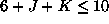and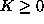.

When the attempt to add a constraint to the store fails, due to inconsistency, a CLP(X) program abandons the current branch of the search tree and tries another choice. In sequential implementations this is usually achieved by backtracking to some previous choice of clause to match with a goal. However there are or-parallel implementations where several branches are explored at the same time, and a failing branch is simply given up, allowing the newly available processor to be assigned to another branch.Next: Constraint Propagation Up: Programming with a Constraint Previous: Primitive Constraints

Mark Wallace
Wed Sep 3 18:36:40 BST 1997